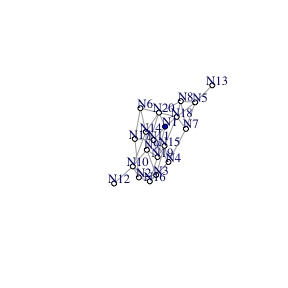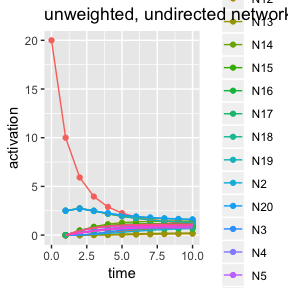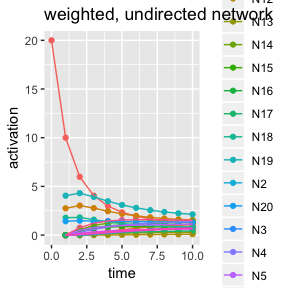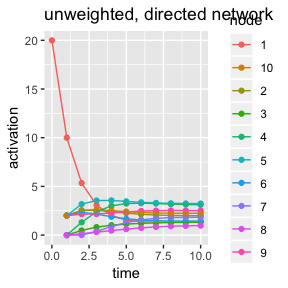Introduction

The notion of spreading activation is a prevalent metaphor in the cognitive sciences; however, the tools to implement spreading activation in a computational simulation are not as readily available. This paper introduces the spreadr R package (pronunced ‘SPREAD-er’), which can implement spreading activation within a specified network structure. The algorithmic method implemented in spreadr subroutines followed the approach described in Vitevitch, Ercal, and Adagarla (2011), who viewed activation as a fixed cognitive resource that could “spread” among connected nodes in a network. See Vitevitch et al. (2011) for more details on the implementation of the spreading activation process.

0: Set up

options(stringsAsFactors = FALSE) # to prevent strings from being converted to a factor class

# install.packages('devtools')
# library(devtools)

1: Generate a network object for spreading activation to occur in

First, the network on which the spreading of activation occurs on must be specified. In this example, we use the sample_gnp function from the igraph R package to generate a network with 20 nodes and undirected links are randomly placed between the nodes with a probability of 0.2. In this step it is important that the network argument in the spreadr function is either (i) recognized by igraph as a network object and has a name attribute (to specify meaningful node labels), or (ii) an adjacency matrix (node names will simply be the column numbers).

Note that in this example spreadr implements spreading activation process in an unweighted, undirected network, but it is possible to implement the process in a weighted network as well whereby more activation is passed between nodes that have “stronger” edges, or in a directed (asymmetric) network where activation can pass from node i to node j but not necessarily from node j to node i (additional examples below).

library(igraph)
##
## Attaching package: 'igraph'
## The following objects are masked from 'package:stats':
##
##     decompose, spectrum
## The following object is masked from 'package:base':
##
##     union
set.seed(1)

g <- sample_gnp(20, 0.2, directed = F, loops = F) # make a random network
V(g)$name <- paste0('N', as.character(1:gorder(g))) # give some meaningful labels for the 'name' attribute V(g)$color <- c('blue', rep('white', 19))
l <- layout_with_fr(g) # to save the layout
plot(g, l = l, vertex.size = 10, vertex.label.dist = 2, vertex.label.cex = 0.8)# the blue node will be assigned activation at t = 0

2: Create a dataframe with initial activation values

The user must then specify the initial activation level(s) of node(s) in the network in a dataframe object with two columns labeled node and activation. Below the node labeled “N1” was assigned 20 units of activation. The user can choose to provide different activation values, or initialize more nodes with various activation values.

initial_df <- data.frame(node = 'N1', activation = 20, stringsAsFactors = F)
initial_df
##   node activation
## 1   N1         20

3: Run the simulation

We are finally ready to run the simulation. In this step, the user must specify the following arguments and parameters in the spreadr function:

1. start_run: the dataframe (initial_df) specified in the previous step that contains the activation values assigned to nodes at t = 0;
2. decay, d: the proportion of activation lost at each time step (range from 0 to 1);
3. retention, r: the proportion of activation retained in the originator node (range from 0 to 1);
4. suppress, d: nodes with activation values lower than this value will have their activations forced to 0. Typically this will be a very small value (e.g., < 0.001);
5. network: the network (must be an igraph object or a non-zero matrix) on which the spreading of activation occurs on, and
6. time, t: the number of times to run the spreading activation process for.
7. create_name: creates numbers/names for nodes if needed, default is TRUE.
retention = 0.5, suppress = 0,
network = g, time = 10)

4: Results

The output is a dataframe with 3 columns labeled node, activation, and time, and contains the activation value of each node at each time step. The output can be easily saved as a .csv file for further analysis later. A plot showing the activation levels of each node in the network at each time step is shown below.

head(result, 10) # view the results
##    node activation time
## 1    N1         10    1
## 2    N2          0    1
## 3    N3          0    1
## 4    N4          0    1
## 5    N5          0    1
## 6    N6          0    1
## 7    N7          0    1
## 8    N8          0    1
## 9    N9          0    1
## 10  N10          0    1
tail(result, 10)
##     node activation time
## 191  N11  1.3281320   10
## 192  N12  0.1420159   10
## 193  N13  0.2170434   10
## 194  N14  1.4254933   10
## 195  N15  1.1792312   10
## 196  N16  0.9448024   10
## 197  N17  0.6828021   10
## 198  N18  1.5931093   10
## 199  N19  1.2969978   10
## 200  N20  1.6084249   10
# write.csv(result, file = 'result.csv') # save the results

library(ggplot2)
a1 <- data.frame(node = 'N1', activation = 20, time = 0) # add back initial activation at t = 0
result_t0 <- rbind(result,a1)
ggplot(data = result_t0, aes(x = time, y = activation, color = node, group = node)) +
geom_point() + geom_line() + ggtitle('unweighted, undirected network') # visualize the resultsThis section shows how spreadr can be implemented in a weighted network (i.e., the edges in the network can have different weights) and a directed network (i.e., the edges in the network are not symmetric).

1. Weighted, undirected network
# weighted, undirected network example
g_w <- g
set.seed(2)
E(g_w)\$weight <- runif(gsize(g_w)) # make the edges in the network have different weights ranging from 0 to 1 (excluding extreme values)

retention = 0.5, suppress = 0,
network = g_w, time = 10)

result_w_t0 <- rbind(result_w, a1) # add back initial activation at t = 0
ggplot(data = result_w_t0, aes(x = time, y = activation, color = node, group = node)) +
geom_point() + geom_line() + ggtitle('weighted, undirected network') # visualize the resultsAs you can see from the plot, the result is slightly different from the original example with unweighted edges. More activation is passed to the node that is more “strongly” connected to the originator node.

1. Unweighted, directed network

For this example, we will create the network via an adjacency matrix.

# unweighted, directed network example
set.seed(3)
g_d_mat <- matrix(sample(c(0,1), 100, replace = T), 10, 10) # make a matrix and randomly fill some cells with 1s
diag(g_d_mat) <- 0 # remove self-loops
g_d_mat
##       [,1] [,2] [,3] [,4] [,5] [,6] [,7] [,8] [,9] [,10]
##  [1,]    0    1    0    0    0    0    1    1    1     0
##  [2,]    1    0    0    0    1    0    0    1    1     0
##  [3,]    0    1    0    0    0    1    1    1    0     0
##  [4,]    0    1    0    0    1    1    0    1    0     1
##  [5,]    1    1    0    0    0    1    1    1    1     1
##  [6,]    1    1    1    0    0    0    0    1    1     0
##  [7,]    0    0    1    1    0    0    0    0    0     0
##  [8,]    0    1    1    0    0    0    1    0    0     0
##  [9,]    1    1    1    1    0    0    0    1    0     0
## [10,]    1    0    1    0    1    0    0    1    1     0
result_d <- spreadr::spreadr(start_run = data.frame(node = 1, activation = 20, stringsAsFactors = F),
decay = 0,
retention = 0.5, suppress = 0,
network = g_d_mat, time = 10)

result_d_t0 <- rbind(result_d, data.frame(node = 1, activation = 20, time = 0)) # add back initial activation at t = 0
ggplot(data = result_d_t0, aes(x = time, y = activation, color = node, group = node)) +
geom_point() + geom_line() + ggtitle('unweighted, directed network')It is important to keep in mind that the directionality of the edges goes column-wise and not row-wise. In other words, node 1 –> node 2 is represented by filling in the cell in COLUMN 1, ROW 2 of the adjacency matrix. See below and compare it to the plot above.

# first column
data.frame(node = 1:10, edge = g_d_mat[,1])
##    node edge
## 1     1    0
## 2     2    1
## 3     3    0
## 4     4    0
## 5     5    1
## 6     6    1
## 7     7    0
## 8     8    0
## 9     9    1
## 10   10    1
# hence activation is going from node 1 to nodes 3,4,10 - as in the plot

# first row - wrong direction!
data.frame(node = 1:10, edge = g_d_mat[1,])
##    node edge
## 1     1    0
## 2     2    1
## 3     3    0
## 4     4    0
## 5     5    0
## 6     6    0
## 7     7    1
## 8     8    1
## 9     9    1
## 10   10    0
# activation does NOT go from node 1 to nodes 3,4,5,7,8,10